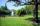# Length + Heron's formula - math problems

#### Number of problems found: 8

• A Cartesian framework1. In a Cartesian framework, the functions f and g we know that: the function (f) is defined by f (x) = 2x ^ 2, the function (g) is defined by g (x) = x + 3, the point (O) is the origin of the reference, point (C) is the point of intersection of the graph
• Vertex pointsGiven the following points of a triangle: P(-12,6), Q(4,0), R(-8,-6). Graph the triangle. Find the triangle area.What is the volume of a quadrilateral oblique prism with base edges of length a = 1m, b = 1.1m, c = 1.2m, d = 0.7m, if a side edge of length h = 3.9m has a deviation from the base of 20° 35' and the edges a, b form an angle of 50.5°.
• Four sides of trapezoidTrapezoid is given by length of four sides: 40.5 42.5 52.8 35.0. Calculate its area.
• ParkIn the newly built park will be permanently placed a rotating sprayer irrigation of lawns. Determine the largest radius of the circle which can irrigate by sprayer P so not to spray park visitors on line AB. Distance AB = 55 m, AP = 36 m and BP = 28 m.
• PrismCalculate the volume of the rhombic prism. The prism base is a rhombus whose one diagonal is 47 cm, and the edge of the base is 28 cm. The edge length of the base of the prism and height is 3:5.
• Triangle ABCCalculate the sides of triangle ABC with area 1404 cm2 and if a: b: c = 12:7:18
• DescribedCalculate perimeter of the circle described by a triangle with sides 478, 255, 352.

We apologize, but in this category are not a lot of examples.
Do you have an exciting math question or word problem that you can't solve? Ask a question or post a math problem, and we can try to solve it.

We will send a solution to your e-mail address. Solved examples are also published here. Please enter the e-mail correctly and check whether you don't have a full mailbox.

Do you want to convert length units? See also our trigonometric triangle calculator. Length - math problems. Heron's formula - math problems.